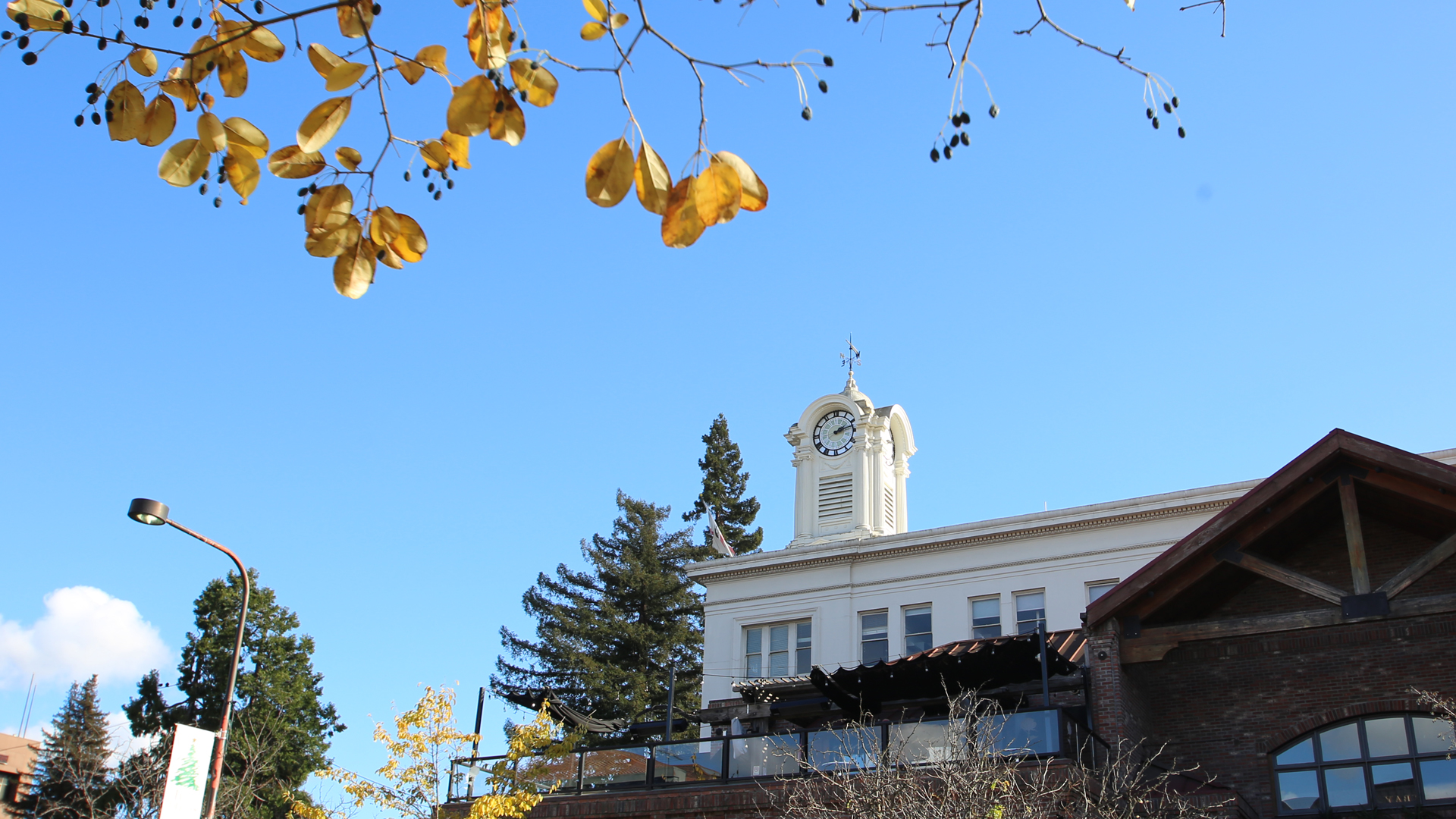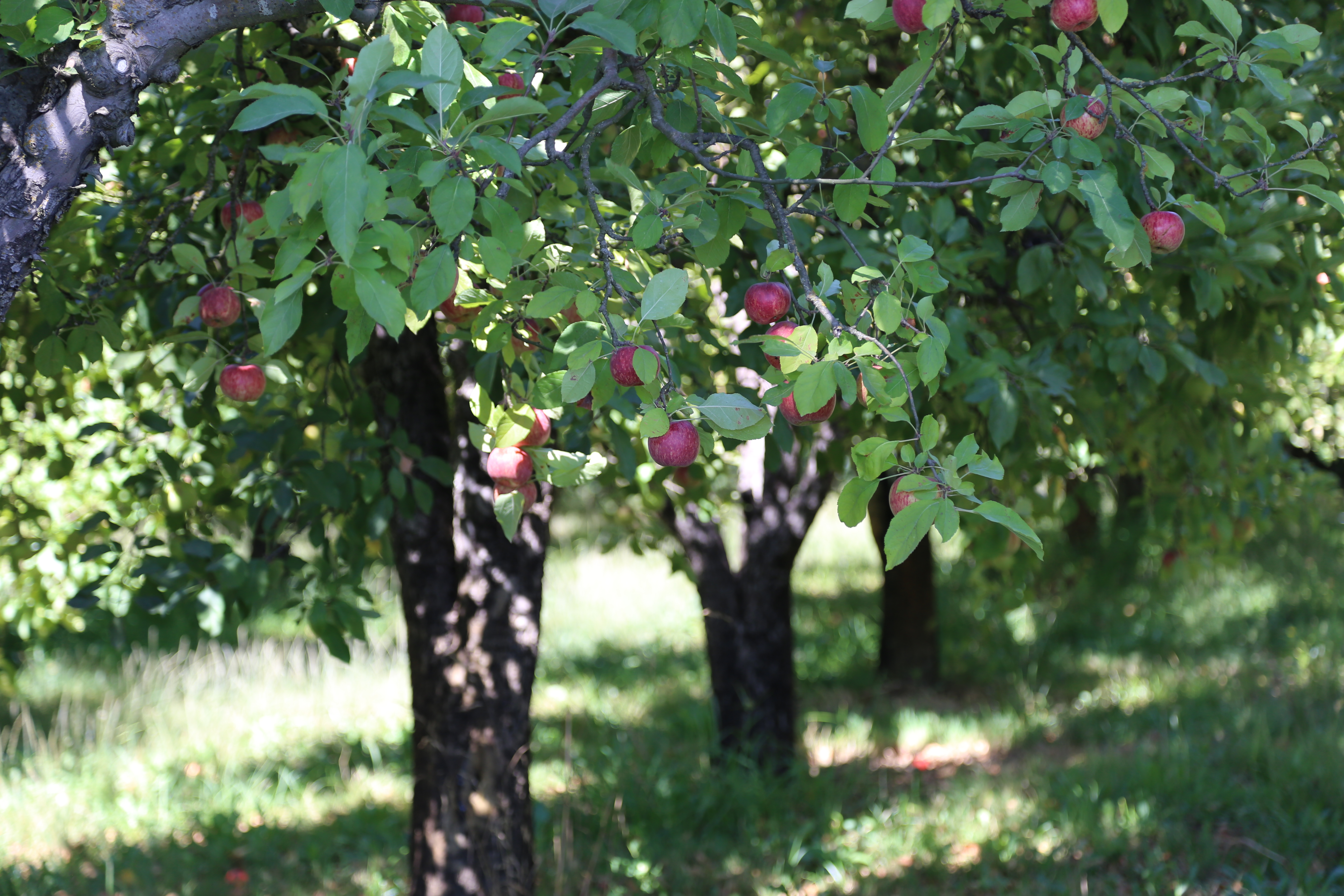Alfred Ramirez - 831.443.1515## Santa Rosa

Welcome to Santa Rosa... This is where the introduction paragraph goes. This is where the introduction paragraph goes. This is where the introduction paragraph goes.## Sonoma

Welcome to Sonoma Valley... This is where the introduction paragraph goes. This is where the introduction paragraph goes. This is where the introduction paragraph goes.## Lake County

Welcome to Lake County... This is where the introduction paragraph goes. This is where the introduction paragraph goes. This is where the introduction paragraph goes.